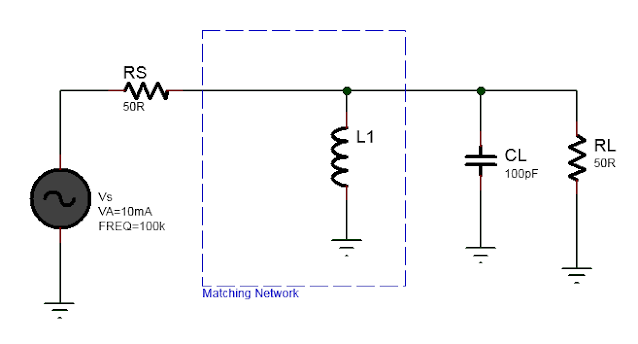# Impedance Matching with parasitic capacitor at the load

Here it is shown how to match source and load with equal resistance but with parasitic capacitor at the load. The parasitic capacitor at the load can be series or parallel. In this case, since source and load resistance are equal we need to add reactance that is opposite in phase but equal in magnitude to match the parasitic capacitor effect. This impedance matching means that maximum power transfer from source to load takes place. The objective to nullify the capacitive effect by adding an inductor in series or parallel that cancels the capacitive reactance effect by the parasitic capacitor.

Consider the following two types of circuits where impedance matching is required.

In the circuit below, the parasitic capacitor at the load is in series with the load.

In the circuit shown below, the parasitic load capacitor is in parallel with the load.

The objective is match the input impedance and the output impedance so that maximum power transfer takes place.

The input impedance or the source impedance for the series load parasitic capacitor circuit is,

$$Z_S = R_S$$

While the output impedance or the load impedance for the series load parasitic capacitor circuit is,

$$Z_L = R_L-jX_C$$

where $$X_C$$ is the capacitive reactance of the series capacitor $$C_L$$

Similarly the input(source) and output(load) impedance of the circuit with parallel parasitic load capacitor are,

$$Z_S = R_S$$ and $$Z_L = R_L+jX_C$$

These two circuit can be impedance matched using an inductor series to the series load parasitic capacitor and adding a parallel inductor in parallel to the parallel load parasitic capacitor as illustrated below.

And,For both the circuit solution presented above, the value of the inductor can be found out using the LC resonant circuit frequency,

$$f=\frac{1}{2\pi \sqrt{LC}}$$

Rearranging for L,

$$L = \frac{1}{(2 \pi f)^2 C}$$

If the design frequency is 100KHz then,

$$L = \frac{1}{(2 \pi 100KHz)^2 100\times 10^{-12} }=25mH$$

Thus the impedance matched circuit solutions for the above two circuits are shown below along with frequency response of the matched circuit.

Series parasitic capacitance at the load

The frequency response graph is shown below,

Parallel parasitic capacitance at the load

The following shows the frequency response of the above matched circuit.

In this way one can compensate energy loss due to parasitic capacitance at the load by inserting an inductor in series or parallel according to the position of the parasitic capacitance. The trick to calculate the inductor value is to think that the parasitic capacitance in circuit can be removed by resonating the circuit. Thus one can use LC resonant frequency to calculate the inductor value.

The impedance problem explained above are useful in impedance matching between circuit or circuit component such as between antenna and RF amplifier, between modulator and RF amplifier in FM transmitter or any circuit where RF signal is flowing.